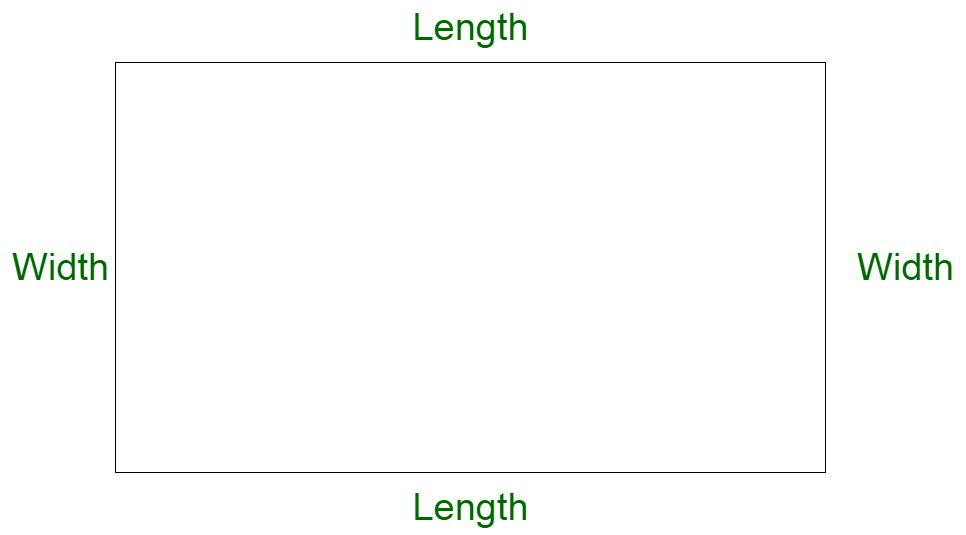# If the length of a rectangle is decreased by 15% and the breadth is increased by 25%, calculate the percentage change in the area

• Last Updated : 24 Feb, 2022

Mensuration is a part or branch of mathematics that deals with the geometric shape calculation and its parameters such as area, length, volume, surface area, etc. It deals with both 2D and 3D shapes. Here 2D shapes are those shapes that only have two dimensions such as length and width but no thickness, or have X and Y dimensions. For example, a square, rectangle, triangle, circle, etc. Whereas 3D shapes are those shapes that have 3 dimensions such as length, width, and thickness, or have X, Y, and Z dimensions. For example, bricks, ice-cream cones, football, etc. Mensuration uses mathematical formulas and algebraic equations to solve problems.

Rectangle

A rectangle is a two-dimensional diagram with four sides. The longer side of the rectangle is the length and the shorter side is called width. It is also known as a quadrilateral with four right angles that is 90° each. If all the sides of a rectangle are equal then the shape is known as a square.Rectangle Properties

The properties of a rectangle are given below:

• In a rectangle, the opposite sides are parallel and equal to each other.
• Both the diagonals of the rectangle have the same length.
• Each interior angle of the rectangle is equal to 90 degrees.
• The sum of all the interior angles of the rectangle is equal to 360 degrees.
• Since the sides of a rectangle are parallel, then it is also known as Parallelogram.
• A rectangle is also known as a quadrilateral with four right angles(each of 90°).
• In a rectangle, both the diagonals always bisect each other.

Rectangle Formulae

Area: The area of the rectangle is known as the area occupied by the rectangle within its boundary.

Area of a Rectangle = (Length × Width) square units.

Perimeter: Perimeter of a rectangle is known as the total distance covered by the sides of the rectangle. So we can say that the perimeter of the rectangle is the sum of its four sides.

Perimeter of a Rectangle Formula = 2 * (Length + Width) units.

Diagonal: A diagonal in a rectangle is a line that joins the opposite vertices. a rectangle contains a maximum of two diagonals and both of them are of equal length.

Diagonal of a Rectangle Formula = √Length2 + Width2 units.

### If the length of a rectangle is decreased by 15% and the breadth is increased by 25%, calculate the percentage change in the area.

Solution:

Step by step explanation of the problem:

Let L, B be length and breadth of rectangle,

then the area of rectangle is

A1 = L * B      …..(1)

Here in the question it’s given that the length decreased by 15% and breadth increased by 25%.

So, new length(L1) = L – L * 15%

= L(1 – 15%)

= L(1 – 0.15)

= L(0.85).

New breadth(B1) = B +  B * 25%

= B(1 + 25%)

= B(1.25).

New Area of rectangle(A2) = L * 0.85 * B * 1.25

= 1.0625LB.

Change in area of rectangles = A2 – A1

= 1.0625LB – LB

= 0.0625LB

= 6.25% of A1. (from eq1)

Percentage change in area of rectangle = change in area / original area

= 6.25 % A1 / A1

= 6.25%.

### Sample Questions

Question 1: The length of a rectangle was decreased by 2% and if breadth is increased by 3% by what percentage did the area of the rectangle change?

Solution:

Percentage Change = l + b + (l * b) / 100

l = -2%, b = 3%

Percentage Change = -2 + 3 + (-2 x 3) / 100 = 1 – 0.06 = 0.94

Positive indicates net increase.

So, the area of rectangle increases by 0.94%.

Question 2: The length of a rectangle was decreased by 35% and if breadth is increased by 25% by what percentage did the area of the rectangle change?

Solution:

Percentage Change = l + b + (l * b) / 100

l = -35%, b = 25%

Percentage Change = -55 + 25 + (-35 x 25) / 100 = -30 – 8.75 = -38.75

Negative indicates net decrease.

So, the area of rectangle decrease by 38.75%.

Question 3: The length of a rectangle was decreased by 1% and if breadth is increased by 1% by what percentage did the area of the rectangle change?

Solution:

Percentage Change = l + b + (l * b)/100

l = -1%, b = 1%

Percentage Change = -1 + 1 + (-1 x 1)/100 = -0.01

Negative indicates net decrease.

So, the area of rectangle decreases by 0.01%.

Question 4: The width of a rectangle was increased by 10%  by what percentage did the area of the rectangle change?

Solution:

Percentage Change = l + b + (l * b)/100

l = 0%, b = 10%

Percentage Change = 0 + 10 + (0 x 10)/100 = 10

Positive indicates net increase.

So, the area of rectangle increases by 10%.

My Personal Notes arrow_drop_up